Olympiad Test: Geometrical Shapes And Angles - 2

# Olympiad Test: Geometrical Shapes And Angles - 2

Test Description

## 10 Questions MCQ Test National Cyber Olympiad Class 5 | Olympiad Test: Geometrical Shapes And Angles - 2

Olympiad Test: Geometrical Shapes And Angles - 2 for Class 5 2023 is part of National Cyber Olympiad Class 5 preparation. The Olympiad Test: Geometrical Shapes And Angles - 2 questions and answers have been prepared according to the Class 5 exam syllabus.The Olympiad Test: Geometrical Shapes And Angles - 2 MCQs are made for Class 5 2023 Exam. Find important definitions, questions, notes, meanings, examples, exercises, MCQs and online tests for Olympiad Test: Geometrical Shapes And Angles - 2 below.
 1 Crore+ students have signed up on EduRev. Have you?
Olympiad Test: Geometrical Shapes And Angles - 2 - Question 1

### Which one of the following angles is obtuse?

Detailed Solution for Olympiad Test: Geometrical Shapes And Angles - 2 - Question 1

Half a right angle = 45°
One right angle = 90°
One and a half right angles = 1½ × 90° = 135°
Two right angles = 180°
Only 135° is greater than 90° but less than 180°, so it is the only obtuse angle.

Olympiad Test: Geometrical Shapes And Angles - 2 - Question 2

### How many straight angles are there in three full rotations?

Detailed Solution for Olympiad Test: Geometrical Shapes And Angles - 2 - Question 2

A full rotation consists of two straight (180°) angles. So three full rotations consist of six straight angles.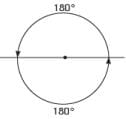Olympiad Test: Geometrical Shapes And Angles - 2 - Question 3

### Which one of the following angles is not reflex?

Detailed Solution for Olympiad Test: Geometrical Shapes And Angles - 2 - Question 3

A reflex angle is more than 180° but less than 360°. 178° is less than 180°, so it is not reflex. (All the rest are reflex angles.)

Olympiad Test: Geometrical Shapes And Angles - 2 - Question 4

Which one of the following angles is reflex?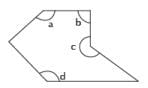Detailed Solution for Olympiad Test: Geometrical Shapes And Angles - 2 - Question 4

A Reflex Angle is greater than 180° but less than 360°. A, B and D are all less than 180°.  Only C is greater than 180° but less than 360°.

Olympiad Test: Geometrical Shapes And Angles - 2 - Question 5

If angle AOB = 67°, what is the size of reflex angle AOB?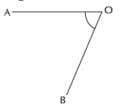Detailed Solution for Olympiad Test: Geometrical Shapes And Angles - 2 - Question 5

If you add the smaller angle (acute or obtuse) and the reflex angle for the same shape you will always come to 360°. Therefore, reflex angle AOB = 360° − 67° = 293°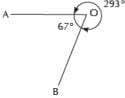Olympiad Test: Geometrical Shapes And Angles - 2 - Question 6

What is the least number of 33° angles you would need to make a reflex angle?

Detailed Solution for Olympiad Test: Geometrical Shapes And Angles - 2 - Question 6

2 × 33° = 66°, which is between 0° and 90°, so acute.
5 × 33° = 165°, which is between 90° and 180°, so obtuse.
6 × 33° = 198°, which is between 180° and 360°, so reflex.
7 × 33° = 231°, which is also reflex, but we have already found the least amount which is 6.

Olympiad Test: Geometrical Shapes And Angles - 2 - Question 7

Which of the following describes the triangle shown above?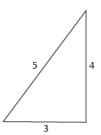Detailed Solution for Olympiad Test: Geometrical Shapes And Angles - 2 - Question 7

The triangle has three different lengths of side, so is scalene. The triangle has lengths 3, 4 and 5 and 52 = 32 + 42, so is right angled. Therefore, it is a scalene right angled triangle.

Olympiad Test: Geometrical Shapes And Angles - 2 - Question 8

A triangle is isosceles and right angled. Which of the following statements must be false?

Detailed Solution for Olympiad Test: Geometrical Shapes And Angles - 2 - Question 8

Since the triangle is isosceles, it must have two equal angles. Since it is also right angled, the other two angles must both be 45° (45° + 45° +90° = 180°). So A is true.
Since the triangle is isosceles, it must have two of its sides equal.
So B is true.
An isosceles triangle has one line of symmetry.
So C is also true.
A triangle that satisfies A, B and C is shown in the following diagram: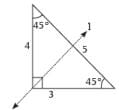Line l is the axis of symmetry
Statement D must be false since a triangle with three different sides cannot be isosceles.

Olympiad Test: Geometrical Shapes And Angles - 2 - Question 9

Shraddha made two copies of the right angled triangle shown and cut them out.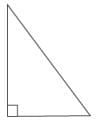Q. She then joined edges of the triangles together to make shapes.  Which of the following shapes was it not possible for Shraddha to make?

Detailed Solution for Olympiad Test: Geometrical Shapes And Angles - 2 - Question 9

Shraddha could make an isosceles triangle, a parallelogram, a rectangle or a kite: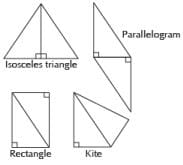But the rectangle is not a square because the sides have different lengths.

Olympiad Test: Geometrical Shapes And Angles - 2 - Question 10

Shraddha made two copies of the following isosceles right angled triangle and cut them out. She then joined edges of the triangles together to make shapes.

Q. Which of the following shapes was it NOT possible for Shraddha to make?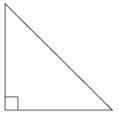Detailed Solution for Olympiad Test: Geometrical Shapes And Angles - 2 - Question 10

Shraddha could make a square, an isosceles triangle (one with 2 equal sides, 2 equal angles), and a parallelogram: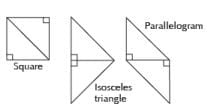But she could not make an equilateral triangle (one with all equal sides, all equal angles).

## National Cyber Olympiad Class 5

1 videos|22 tests
 Use Code STAYHOME200 and get INR 200 additional OFF Use Coupon Code